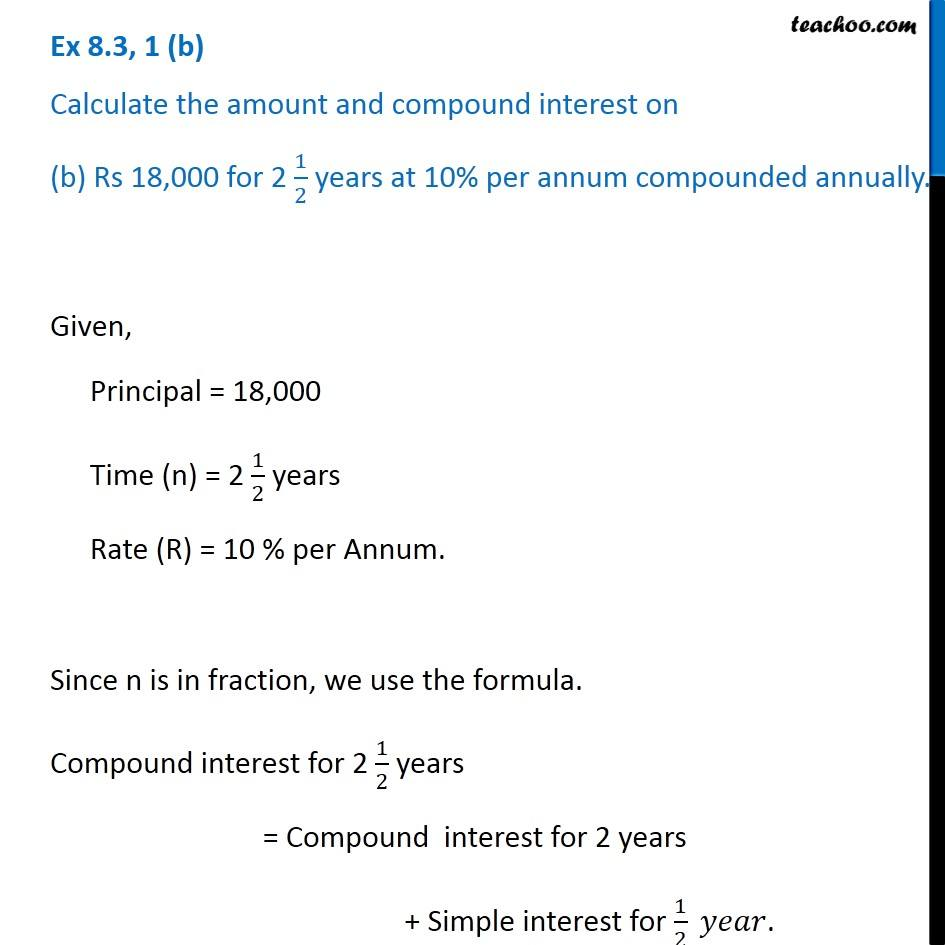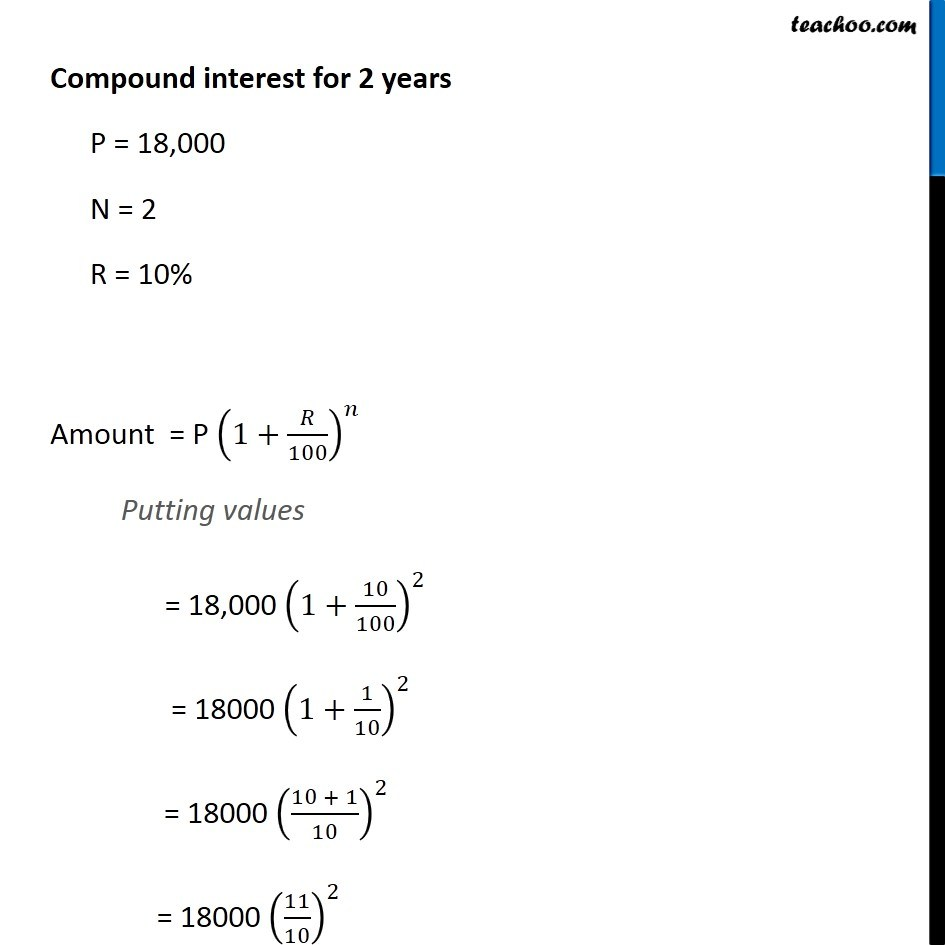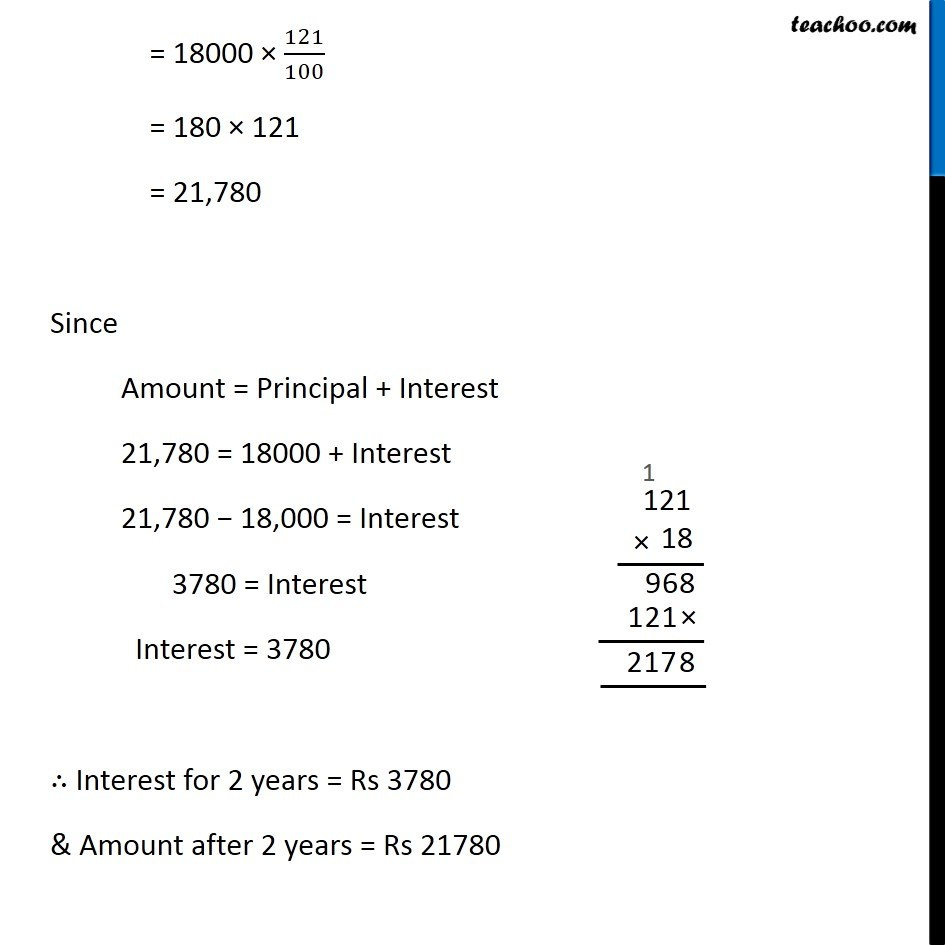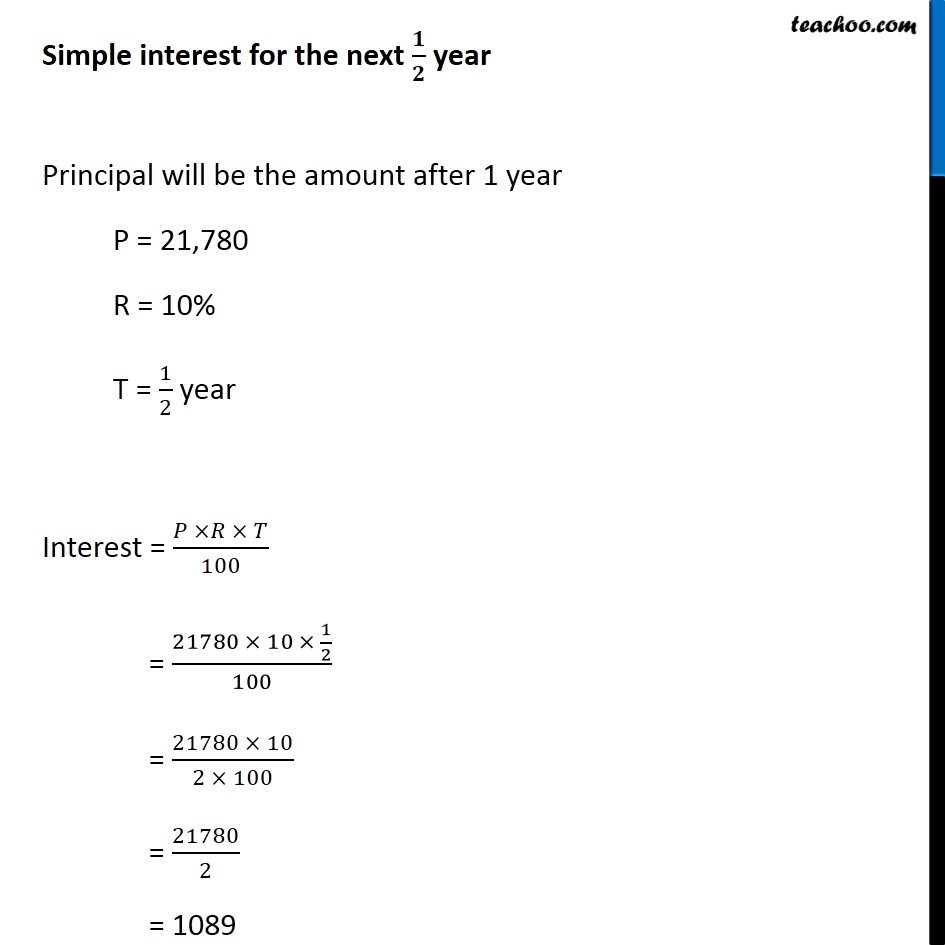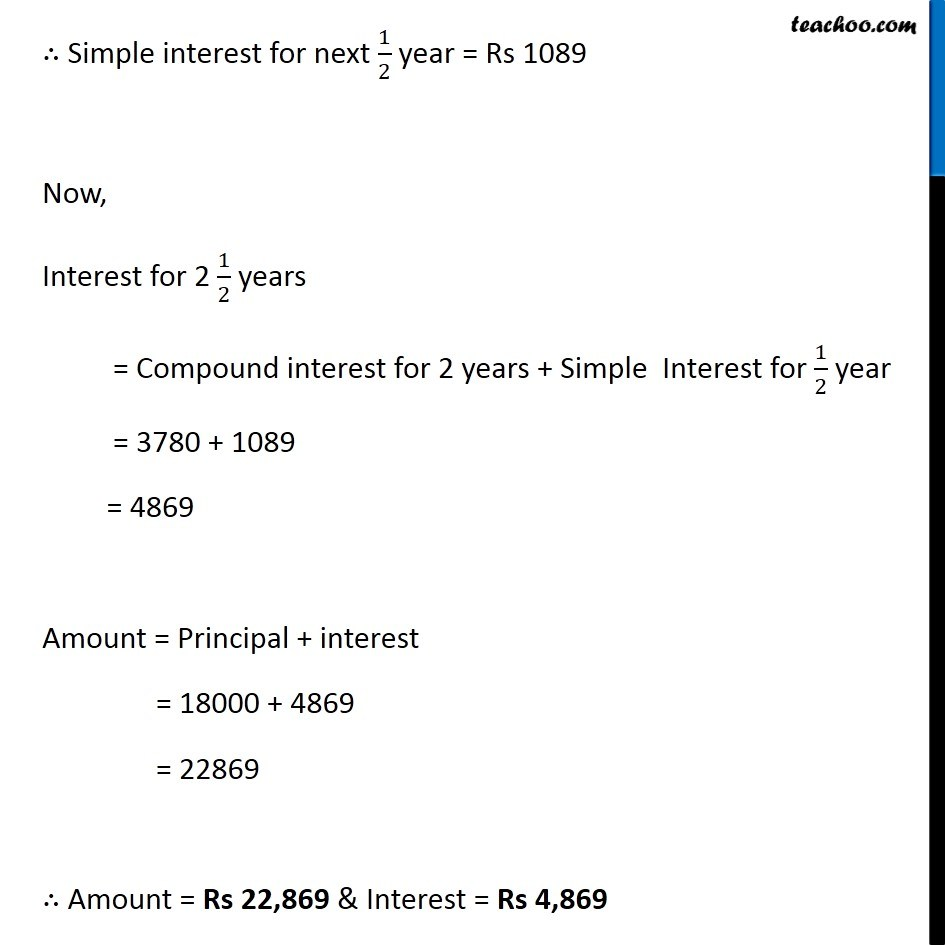Subscribe to our Youtube Channel - https://you.tube/teachoo

1. Chapter 8 Class 8 Comparing Quantities
2. Concept wise
3. Compound Interest for fraction years

Transcript

Ex 8.3, 1 (b) Calculate the amount and compound interest on (b) Rs 18,000 for 2 1/2 years at 10% per annum compounded annually. Given, Principal = 18,000 Time (n) = 2 1/2 years Rate (R) = 10 % per Annum. Since n is in fraction, we use the formula. Compound interest for 2 1/2 years = Compound interest for 2 years + Simple interest for 1/2 𝑦𝑒𝑎𝑟. Compound interest for 2 years P = 18,000 N = 2 R = 10% Amount = P (1+𝑅/100)^𝑛 Putting values = 18,000 (1+10/100)^2 = 18000 (1+1/10)^2 = 18000 ((10 + 1)/10)^2 = 18000 (11/10)^2 = 18000 × 121/100 = 180 × 121 = 21,780 Since Amount = Principal + Interest 21,780 = 18000 + Interest 21,780 − 18,000 = Interest 3780 = Interest Interest = 3780 ∴ Interest for 2 years = Rs 3780 & Amount after 2 years = Rs 21780 Simple interest for the next 𝟏/𝟐 year Principal will be the amount after 1 year P = 21,780 R = 10% T = 1/2 year Interest = (𝑃 ×𝑅 × 𝑇)/100 = (21780 × 10 × 1/2)/100 = (21780 × 10)/(2 × 100) = 21780/2 = 1089 ∴ Simple interest for next 1/2 year = Rs 1089 Now, Interest for 2 1/2 years = Compound interest for 2 years + Simple Interest for 1/2 year = 3780 + 1089 = 4869 Amount = Principal + interest = 18000 + 4869 = 22869 ∴ Amount = Rs 22,869 & Interest = Rs 4,869

Compound Interest for fraction years